How to convert the output voltage value (V) of the MI-8100 directional microphone into the sound pressure level value (dB).The following explanation is how to convert the output voltage from the SR-2200 into the sound pressure level (dB), when the MI-8100 directional microphone is used with SR-2200 sensor amplifier.

 Measurement condition   MI-8100 sensitivity: -30.41 dB re1 V/Pa at 1 kHz (From the laboratory data attached to the MI-8100.)   SR-2200 gain setting: 20 dB (-10 to 60 dB, 10 dB-step eight switching)

The output voltage value from the SR-2200 is proportional to the sound pressure (= Pascal). The sound pressure level (dB) is logarithmic value, so when the voltage value is converted into sound pressure level, use the calculator and process the logarithmic calculation.

The sound pressure level (dB) is effective value. On the other hand, the time waveform which is outputted by the MI-8100 via SR-2200 is not effective value but instantaneous value. Therefore, value which converts the output voltage from the SR-2200 into sound pressure level (dB) is considered to be a reference value. In addition, when the voltage output from the SR-2200 are analyzed in the FFT analyzer, Y axis of FFT is displayed to be dB effective value, and the signal is correctly displayed as the pressure level (dB) by using the unit calibration function.

The MI-8100 is directional microphone, so the measurement results are different from the non-directional microphone such as a sound level meter.

Concept of conversion

When the MI-8100 is used with the SR-2200, conversion from output voltage (V) to sound pressure level (dB) is considered as follows. The "r" of "Vr" means effective value in the following explanations.

MI-8100 directional microphone sensitivity

When sensitivity of the MI-8100 "-30.41 dB re.1 V/Pa at 1 kHz" means "When the frequency is 1 kHz and sound pressure is 1 Pa (sound pressure level: 94 dB), the output voltage becomes -30.41 dBVr (voltage)".

SR-2200 microphone amplifier output voltage

When the gain of the SR-2200 is set as 20 dB, the output voltage of SR-2200 is 20 dB (10 times) amplified voltage of MI-8100. So, when the sound pressure is 1 Pa (sound pressure level: 94 dB), the output voltage is -30.41 + 20 dB = -10.41 dBVr.

When the gain of the SR-2200 is 20 dB, the voltage for sound pressure 1 Pa is -10.41 dBVr. Convert it into the voltage as follows;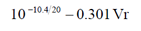Therefore, when frequency is 1 kHz and sound pressure is 1 Pa, the voltage output from the SR-2200 is 0.0301 Vr.

Conversely, when output voltage from the SR-2200 is 1 kHz and 0.301 Vr, conversion into the sound pressure level dB is as follows;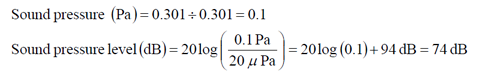When output voltage from the SR-2200 is 1 kHz and 0.0301 Vr, the sound pressure level is 74 dB.

The calculation formula which is organized above results is as follows which is set the gain as G (dB) and output voltage effective value as A (Vr) of the SR-2200.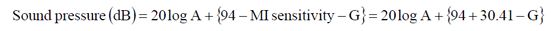(1)

Instantaneous value and effective value

When the waveform is sine wave, the relation of half amplitude V and effective value A (Vr) is as follows;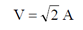When the instantaneous waveform is sine wave, from the formula (1);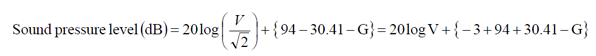When the waveform is sine wave, the sound pressure level dB value can be obtained with the formula (3) by converting half amplitude V which is read from the waveform. However, the voltage which is read from the complicate instantaneous waveform is not effective value, so the conversion into the sound pressure level dB with the formula (3) is considered as [the reference value which is obtained by the formula (3)].

To convert the output voltage from the SR-2200 into the sound pressure level (dB) correctly, we recommend you to use the FFT Analyzer.

Revised:2008.10.20